# (Machine|Statistical) Learning - (Predictor|Feature|Regressor|Characteristic) - (Independent|Explanatory) Variable (X)

A Independent variable is a variable used in supervised analysis in order to predict an outcome variable.

It's also known as:

• Predictor
• Input variable,
• Regressors,
• Manipulated variable,
• Characteristic
• Control variable
• Controlled variable
• Observed variable
• Explanatory variable
• Exposure variable

## Independent variable

In statistics, a predictor is well known as an independent variable (IV). It does not depend of the experimentational procedure and is more generally:

• a description of the characteristics of a group.
• better known as the manipulation, the treatment or conditions.

## Quasi-independent

A Quasi-independent variable is a variable that can not be random assigned (example: concussions, gender, Sexual orientation) .

Since the independent variable does not involve random and representative sampling, arguments about causality are not as strong

## Type

### Categorical

Some predictors are not quantitative but are qualitative, taking a discrete set of values.

These are also called:

• categorical predictors

Example:

• gender,
• student (student status),
• status (marital status)

## Documentation / Reference

Recommended Pages(Machine|Statistical) Learning - (Target|Learned|Outcome|Dependent|Response) (Attribute|Variable) (Y|DV)

An (outcome|dependent) variable is ameasure that we want to predict. : the original score collected : the predicted score (or estimator) from the equation. The hat means “estimated” from the...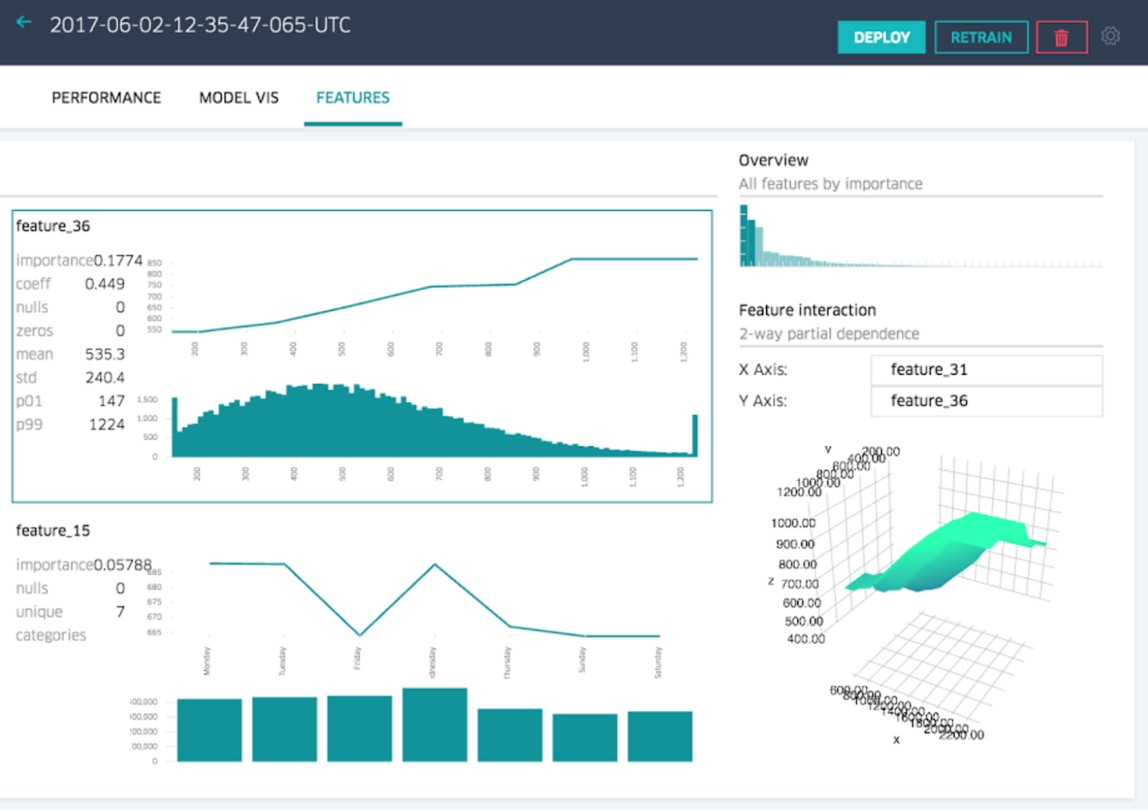Data Mining - (Attribute|Feature) (Selection|Importance)

Feature selection is the second class of dimension reduction methods. They are used to reduce the number of predictors used by a model by selecting the best d predictors among the original p predictors....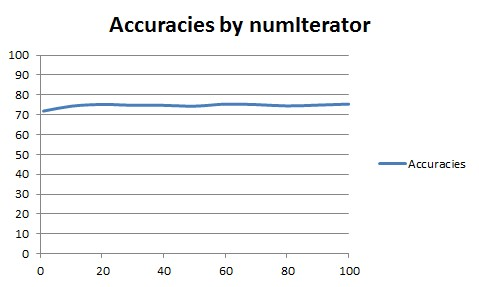Data Mining - (Boosting|Gradient Boosting|Boosting trees)

Boosting forces new classifiers to focus on the errors produced by earlier ones. boosting works by aggressively reducing the training error Gradient Boosting is an algorithm based on an ensemble of decision...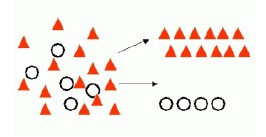Data Mining - (Classifier|Classification Function)

A classifier is a Supervised function (machine learning tool) where the learned (target) attribute is categorical (“nominal”) in order to classify. It is used after the learning process to classify...Data Mining - (Discriminative|conditional) models

Discriminative models, also called conditional models, are a class of models used in machine learning for modeling the dependence of an unobserved variable y on an observed variable x. Discriminative...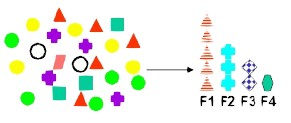Data Mining - (Feature|Attribute) Extraction Function

Feature extraction is the second class of methods for dimension reduction. dimension reduction It creates new attributes (features) using linear combinations of the (original|existing) attributes. ...Data Mining - (Function|Model)

The model is the function, equation, algorithm that predicts an outcome value from one of several predictors. During the training process, the models are build. A model uses a logic and one of several...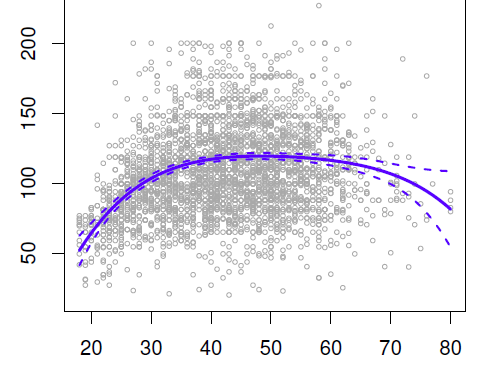Data Mining - (Global) Polynomial Regression (Degree)

polynomials regression Although polynomials are easy to think of, splines are much better behaved and more local. With polynomial regression, you create new variables that are just transformations...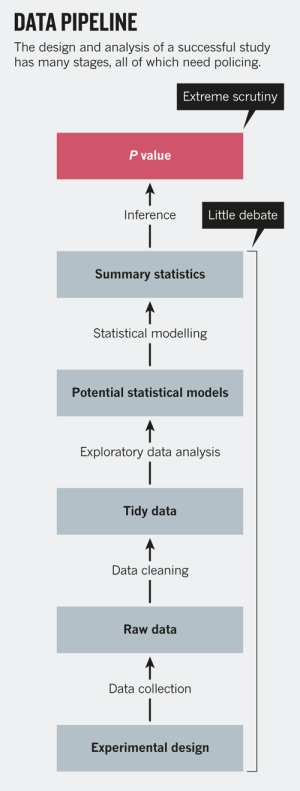Data Mining - (Life cycle|Project|Data Pipeline)

Data mining is an experimental science. Data mining reveals correlation, not causation. With good data, you will make good algorithm. The most preferable solution is then to work on good features....Data Mining - (Stochastic) Gradient descent (SGD)

Gradient descent can be used to train various kinds of regression and classification models. It's an iterative process and therefore is well suited for map reduce process. The gradient descent update...